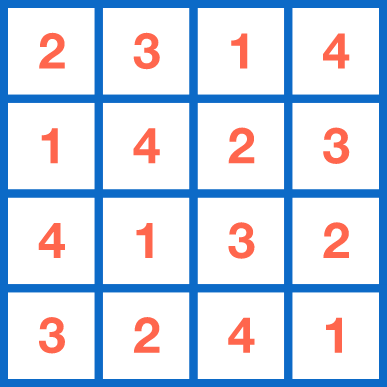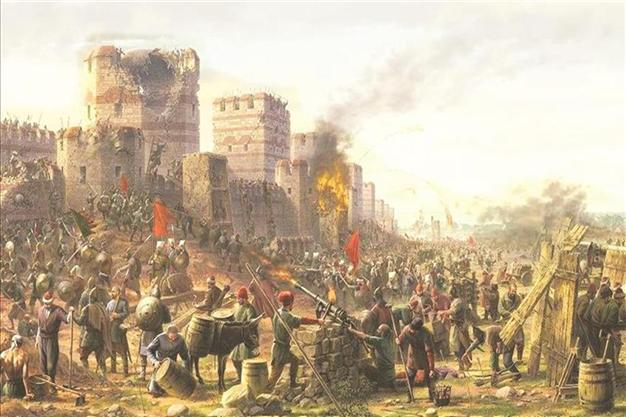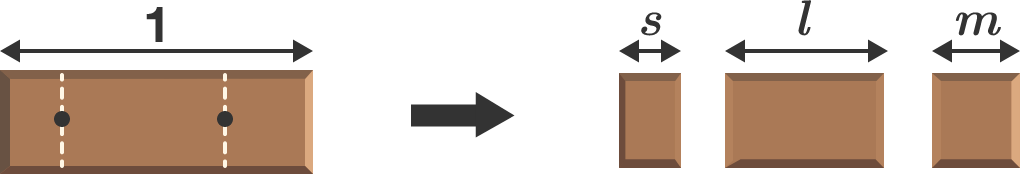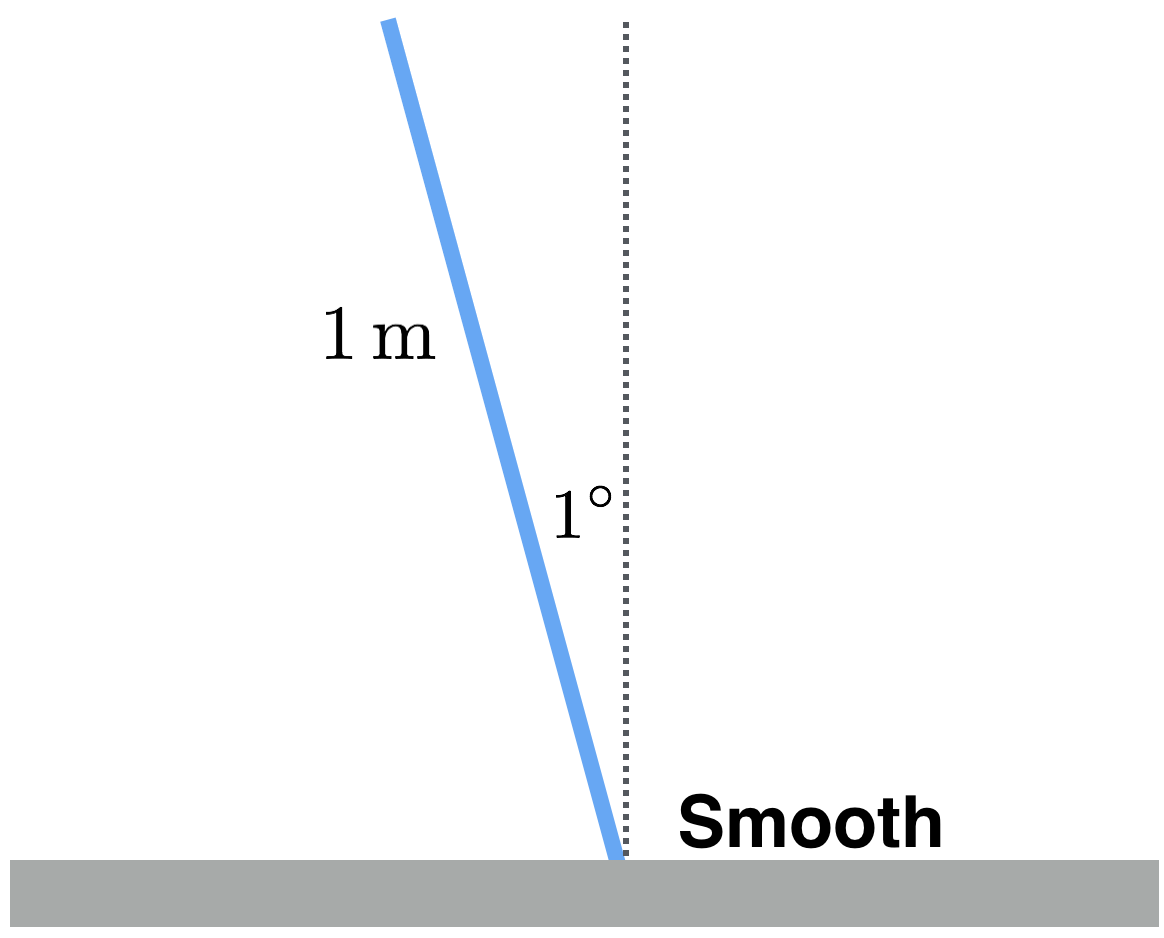# Problems of the Week

Contribute a problemIs it possible to construct an infinite version of the table above (i.e. so that each row and each column contain all positive integers exactly once)?You're a $15^\text{th}$ century ballistics expert serving under the great Ottoman general Sultan Mehmed the Conqueror, tasked with ensuring a solid connection between cannon fire and the heavily fortified city walls of Constantinople.

You're setting up the cannon, which has a muzzle velocity of $v_0 = \SI[per-mode=symbol]{300}{\meter\per\second},$ for a shot at a crucial encampment some $\SI{2000}{\meter}$ away at the heart of the city when a few upstarts in your outfit start yelling at you, upset that you insist upon careful evaluation of the flight path of the cannonball, and urge you to take a more freewheeling approach. Historians will later note a band of Ottomans screaming at a dejected engineer, "just let it rip."

You hold steady and tell them to find something else to do.

Suppose you allowed the yahoos to have their way and ignored the effects of wind drag. By what margin $($in $\si{\meter})$ would your cannon fire undershoot the encampment?

Assume that the force of wind resistance has the form $\mathbf{F}_\textrm{drag} = -\frac12 \rho_\textrm{air}C_d Av^2 \hat{\mathbf{v}},$ where $\hat{\mathbf{v}}$ is a unit vector in the direction of the total velocity $\mathbf{v},$ $A$ is the cross-sectional area of the cannonball, and the constants are as defined in the code box below (in SI units). Moreover, the cannonball is a steel sphere of radius $r$ and density $\rho_\textrm{steel}.$ To avoid collateral damage, you only choose launch angles $\theta \geq \SI{40}{\degree}.$ For historical context, read on the Fall of Constantinople.

import math

rho_air = 1.22
g = 10.0
rho_steel = 8050.0
v0 = 300.0
r = 0.08
theta = # Fill this in.
drag = "Off" # Set this to "On"  or "Off" to include drag, or not.
C_d = (1 if drag is "On" else 0)

(vx, vy) = (v0 * math.cos(theta * math.pi / 180), v0 * math.sin(theta * math.pi / 180))
(x, y) = (0, 0)

dt = 0.001

while y >= 0:
# Finish this code to step through the motion of the cannonball.
(x, y) = (x + ..., y + ...)
(vx, vy) = (vx + ..., vy + ...)

print("The cannonball's range is {:.2f} meters.".format(x))

Python 3
You need to be connected to run code

You have many chocolate bars of unit length and start breaking each of them into 3 pieces by randomly choosing two points on the bar. What are the average lengths of the shortest, medium, and longest pieces?

If the product of these averages can be expressed as $\frac pq$, where $p$ and $q$ are coprime positive integers, give your answer as $p+ q$.A massive, rigid, uniform rod is initially at rest, tilted one degree $(1^\circ)$ away from the vertical. The rod stands on a smooth surface, and its bottom end can slide along the surface.

How many seconds does it take for the rod to become horizontal?


Details and Assumptions:

1. The rod is 1 meter long.
2. There is a downward gravitational acceleration $g = 10\text{ m/s}^2$.
3. While the bottom end of the rod can slide along the surface, it cannot lose contact with it.

$A(x)=x+\cfrac { 1 }{ x+\cfrac { 1 }{ x+\cfrac { 1 }{ \ddots } } }$ With $A(x)$ defined as above, where the continued fraction goes on indefinitely, find the value of the infinite product $\dfrac { 1 }{ A(1) } \times \dfrac { 1+\frac { 1 }{ 1 } }{ A(1) } \times \frac { 1+\frac { 1 }{ 1+\frac { 1 }{ 1 } } }{ A(1) } \times \cdots .$ If your answer can be expressed as $\dfrac { a+\sqrt { b } }{ c\sqrt { b } },$ where $a, b, c$ are positive integers and $b$ is square-free, give your answer as $100a+10b+c$.

Bonus: Can you give a closed formula for $\dfrac { y }{ A(y) } \times \dfrac { y+\frac { 1 }{ y } }{ A(y) } \times \dfrac { y+\frac { 1 }{ y+\frac { 1 }{ y } } }{ A(y) } \times \cdots$ when it converges?

×